# 10 Best Books To Learn MATLAB Programming in 2023

Learning MATLAB seems like a daunting task when you are just a beginner. But with the right guidance, you can excel in just a matter of months. Many prefer referring to YouTube videos when it comes to learning technological things. But there’s no comparison to the old-school book reading.

Books on MATLAB are not just full of theoretical concepts. While compiling our list of best MATLAB books, we took care that ample importance is given to practical applications. With these books, you will not only master the theory but will also effectively put it to use.

## List of Best Books to Learn MATLAB

Preview Product Price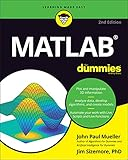MATLAB For Dummies (For Dummies (Computer/Tech)) \$23.23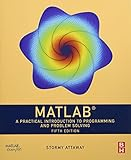MATLAB: A Practical Introduction to Programming and Problem Solving \$31.99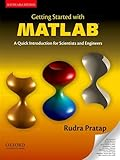Getting Started with MATLAB: A Quick Introduction for Scientists and Engineers \$5.58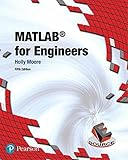MATLAB for Engineers \$16.43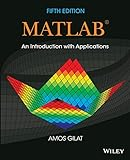MATLAB: An Introduction with Applications \$8.98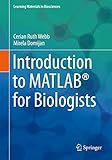Introduction to MATLAB® for Biologists (Learning Materials in Biosciences) \$64.99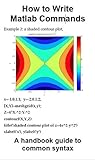How to Write MATLAB Commands: a handbook guide to common syntax \$0.99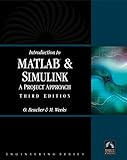Introduction to MATLAB and SIMULINK: A Project Approach \$87.94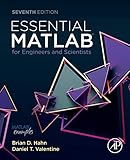Essential MATLAB for Engineers and Scientists \$44.05

## Best for Beginners: MATLAB For Dummies

Ideal for: Absolute beginners

Not ideal for: Engineers

Number of pages: 432

If you are a complete beginner and want to learn MATLAB from scratch then this book is an excellent guide. With this book, you will learn the various syntax and commands used in MATLAB. And by the end of this book, you will successfully be able to analyze data, develop algorithms, and create models.

Key Features:

• Solve complex problems in mathematics with the help of extensive MATLAB functions
• Detailed explanation on how to save, import, export, print, and publish data in MATLAB
• Tricks and tips to automate your data in MATLAB
• Learn to communicate your ideas via plots and graphs which you can generate using MATLAB.

## Best for Programming: MATLAB – Programming and Problem-Solving

Ideal for: Beginners

Not ideal for: Experienced users

Number of pages: 626

Number of chapters: 14

What’s better than learning MATLAB from an award-winning book? This book has received the TAA’s 2017 Textbook Excellence Award. It helps the reader navigate the commands to MATLAB to use it for complex engineering applications. An ample focus is given to programming in MATLAB so that beginners will benefit the most.

Key Features:

• Starts with very basics like variables, assignments, selection statements, and loops
• No need to have any prior knowledge of programming
• Teaches how to import data from websites to use it for further programming
• Numerous solved examples and practice questions test the reader’s understanding of concepts and help build up patience which is vital with MATLAB programming

## Best for Engineers (Begineer-Oriented): MATLAB for Scientists and Engineers

Ideal for: Scientists and Engineers

Number of pages: 230

If you are starting your career in engineering then this book will be a good guide in covering the engineering-oriented basics of MATLAB. It covers elementary, advanced, as well as special functions of MATLAB. With the help of figures, graphs, and commands, the author teaches you to gain a strong hold on MATLAB.

Key Features:

• It’s an interactive book that teaches via examples so you can put the knowledge gained into practice
• Full of appealing illustrations that depict the computer screen gives you an idea about how certain functions look like
• 2 sets of self-guided tutorials to show essential features of MATLAB
• The publisher also provides extra online assistance with the latest updates in MATLAB software

## Best for Engineers: MATLAB for Engineers

Ideal for: Engineers

Not ideal for: School students

Number of pages: 688

Owing to the author’s significant teaching experience, this book is perfect for engineers. The author uses very simple language, excellent illustrations, and significant examples to get readers well acquainted with MATLAB programming. The book starts with the basics of algebra, so you don’t have to look anywhere else to learn to use MATLAB.

Key Features:

• Includes mathematical concepts of algebra, trigonometry, statistics, matrices, calculus, differential equations, etc
• Emphasis is given on example-based learning and practical scenarios
• Examples based on concepts in physics and chemistry making it ideal for students getting a bachelor’s degree in science
• Covers even the esoteric commands and functions which are usually not covered in many books

## Best for Applications: MATLAB Applications

Ideal for: Beginners

Number of pages: 414

This book is loved by many students for being an ideal, concise textbook on MATLAB. Solved examples based on applications in the field of mathematics, physics, and chemistry will help you understand how to use MATLAB for various fields. This highly illustrative book serves as an excellent self-learning guide for beginners and as a refresher course for seasoned users.

Key Features:

• Generous amounts of computer screenshots for better understanding
• Step-by-step tutorials in most sections make your learning easier
• It covers abundant applications in the fields of science, mathematics, and engineering
• Teaches how to make 2D and 3D graphics

## Best for Biologists: MATLAB for Biologists

Ideal for: Researchers

Not ideal for: Readers who don’t want biology-based examples

Number of pages: 283

Number of chapters: 11

This book is ideal for students pursuing their career in biosciences. It will teach you everything from using the interface to creating your own scripts and functions. The examples in this book are relevant to biological studies, but that should not prevent other learners from learning the basics of MATLAB.

Key Features:

• The ultimate aim of this book is to help biologists use MATLAB to support their research
• This book not only just reached to use MATLAB but also explains how to debug certain common errors
• Touches upon how MATLAB can be used as a programming language using loops and conditional statements

## Best for Writing Commands: How to Write MATLAB Commands

Ideal for: MATLAB users

Not ideal for: Beginners

Number of pages: 92

Number of chapters: 16

This booklet is completely dedicated to writing syntax in MATLAB. It is a short handbook that focuses on all aspects of syntax writing in MATLAB. It will come in handy for someone who regularly uses MATLAB. But if you want to start learning MATLAB from scratch then this is not the book for you.

Key Features:

• Everything to know about syntax and commands in MATLAB is gathered in a single place
• Commands are grouped together into hyperlinked sections
• Explanations are delivered via examples that can be modified to suit your needs
• Information is sorted into neat sections which makes the booklet easy to navigate

## Introduction to MATLAB and SIMULINK: A Project Approach

Ideal for: Beginners

Not ideal for: Regular users

Number of pages: 386

Number of chapters: 4

This is a combined book on MATLAB and SIMULINK. You can learn modeling with both of these platforms. You will require a basic understanding of mathematics and physics to start this book. With this book, you will learn to successfully simulate dynamic systems in the field of mathematics, engineering, and sciences.

Key Features:

• 20 hands-on projects that provide a practical mastery of the subject areas
• 80+ in-text exercises to test your understanding of the subject matter
• It comes with a CD-ROM full of source codes
• Complete solutions to MATLAB and SIMULINK problems in separate sections available at the end of the book

Check also: 15 Best C Programming Books

## Essential MATLAB for Engineers and Scientists

Ideal for: Beginners

Not ideal for: Researchers

Number of pages: 428

Number of chapters: 17

If it’s your first time learning MATLAB programming then this book will help you a long way. It is designed in a way to introduce newbies to vital fundamentals of MATLAB and then use them in practice. Even though the title suggests that this book is for engineers and scientists, you can use it to get acquainted with the basics.

Key Features:

• Complete coverage on windows and menus of MATLAB software
• Emphasis on algorithm development and program design
• Dedicated chapter on toolboxes
• Wide range of examples of engineering and scientific areas

## MATLAB for Beginners: A Gentle Approach

No products found.

Ideal for: High school students

Number of pages: 288

Number of chapters: 11

As the title suggests, this book is perfect for beginners with no prior knowledge of MATLAB. It contains examples from multiple fields, including mathematics, engineering, physics, biology, environmental science, etc. It introduces students to the simple but powerful computational abilities of MATLAB software.

Key Features:

• Major topics covered are arithmetic operations, variables, mathematical functions, complex numbers, vectors, matrices, programming, graphs, solving equations, and an introduction to calculus
• Emphasis on MATLAB Symbolic Math Toolbox
• 230+ end-of-chapter exercises for extra practice
• Solutions with detailed explanations to all questions available in the second half of the book

## Conclusion: Best Books To Learn MATLAB Programming

These MATLAB books will help you in understanding the different concepts in programming and syntax. Some of them even offer additional MATLAB resources that will help you throughout your lifetime. All books have a unique perspective and different degrees of knowledge.

If you are an absolute beginner, then we would suggest you MATLAB for Dummies. Otherwise, you can go for an advanced or field-specific book.